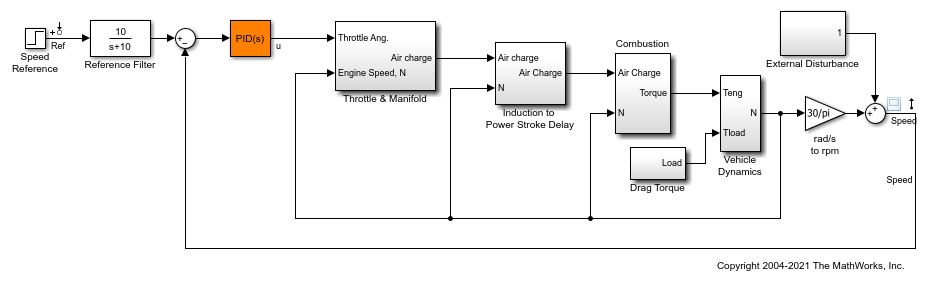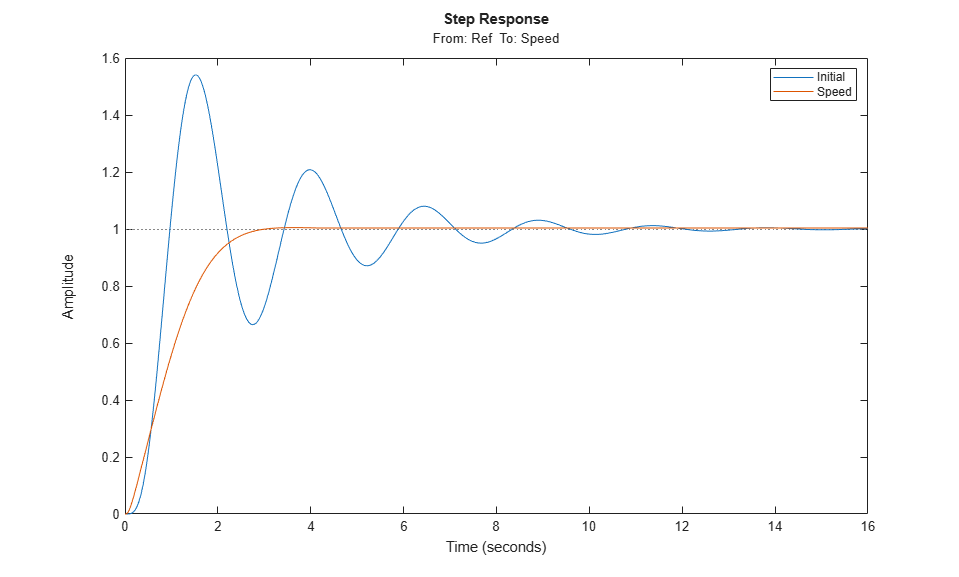# looptune

Tune MIMO feedback loops in Simulink using `slTuner` interface

## Syntax

``````[st,gam,info] = looptune(st0,controls,measurements,wc)``````
``````[st,gam,info] = looptune(st0,controls,measurements,wc,req1,...,reqN)``````
``````[st,gam,info] = looptune(___,opt)``````

## Description

example

``````[st,gam,info] = looptune(st0,controls,measurements,wc)``` tunes the free parameters of the control system of the Simulink® model associated with the `slTuner` interface, `st0`, to achieve the following goals:Bandwidth — Gain crossover for each loop falls in the frequency interval `wc`Performance — Integral action at frequencies below `wc`Robustness — Adequate stability margins and gain roll-off at frequencies above `wc``controls` and `measurements` specify the controller output signals and measurement signals that are subject to the goals, respectively. `st` is the updated `slTuner` interface, `gam` indicates the measure of success in satisfying the goals, and `info` gives details regarding the optimization run.Tuning is performed at the sample time specified by the `Ts` property of `st0`. For tuning algorithm details, see Algorithms. ```
``````[st,gam,info] = looptune(st0,controls,measurements,wc,req1,...,reqN)``` tunes the feedback loop to meet additional goals specified in one or more tuning goal objects, `req`. Omit `wc` to drop the default loop shaping goal associated with `wc`. Note that the stability margin goals remain in force.```
``````[st,gam,info] = looptune(___,opt)``` specifies further options, including target gain and phase margins, number of runs, and computation options for the tuning algorithm. Use `looptuneOptions` to create `opt`.If you specify multiple runs using the `RandomStarts` property of `opt`, `looptune` performs only as many runs required to achieve the target objective value of 1. Note that all tuning goals should be normalized so that a maximum value of 1 means that all design goals are met.```

## Examples

collapse all

Tune the PID Controller in the `rct_engine_speed` model to achieve the specified bandwidth.

```mdl = 'rct_engine_speed'; open_system(mdl); ```Create an `slTuner` interface for the model.

```st0 = slTuner(mdl,'PID Controller'); ```

Add the PID Controller output, `u`, as an analysis point to `st0`.

```addPoint(st0,'u'); ```

Based on first-order characteristics, the crossover frequency should exceed 1 rad/s for the closed-loop response to settle in less than 5 seconds. So, tune the PID loop using 1 rad/s as the target 0 dB crossover frequency.

```wc = 1; st = looptune(st0,'u','Speed',wc); ```
```Final: Peak gain = 0.979, Iterations = 4 Achieved target gain value TargetGain=1. ```

In the call to `looptune`, `'u'` specifies the control signal, and `'Speed'` specifies the measured signal.

Compare the tuned and initial response.

```stepplot(getIOTransfer(st0,'Ref','Speed'),getIOTransfer(st,'Ref','Speed')); legend('Initial','Speed'); ```View the tuned block value.

```showTunable(st) ```
```Block 1: rct_engine_speed/PID Controller = 1 s Kp + Ki * --- + Kd * -------- s Tf*s+1 with Kp = 0.00062, Ki = 0.00303, Kd = 0.000168, Tf = 0.01 Name: PID_Controller Continuous-time PIDF controller in parallel form. ```

To write the tuned values back to the Simulink model, use `writeBlockValue`.

## Input Arguments

collapse all

Interface for tuning control systems modeled in Simulink, specified as an `slTuner` interface.

Controller output name, specified as one of the following:

• Character vector — Name of an analysis point of `st0`.

You can specify the full name or any portion of the name that uniquely identifies the analysis point among the other analysis points of `st0`.

For example, `'u'`.

• Cell array of character vectors — Multiple analysis point names.

For example, `{'u','y'}`.

Measurement signal name, specified as one of the following:

• Character vector — Name of an analysis point of `st0`.

You can specify the full name or any portion of the name that uniquely identifies the analysis point among the other analysis points of `st0`.

For example, `'u'`.

• Cell array of character vector — Multiple analysis point names.

For example, `{'u','y'}`.

Target crossover region, specified as one of the following:

• `[wcmin,wcmax]``looptune` attempts to tune all loops in the control system so that the open-loop gain crosses 0 dB within the target crossover region.

• Positive scalar — Specifies the target crossover region as `[wc/10^0.1,wc*10^0.1]` or `wc` +/- 0.1 decades.

Specify `wc` in the working time units, that is, the time units of the model.

Design goals, specified as one or more `TuningGoal` objects.

For a complete list of the design goals you can specify, see Tuning Goals (Control System Toolbox).

Tuning algorithm options, specified as an options set created using `looptuneOptions`.

Available options include:

• Number of additional optimizations to run starting from random initial values of the free parameters

• Tolerance for terminating the optimization

• Flag for using parallel processing

• Specification of target gain and phase margin

## Output Arguments

collapse all

Tuned interface, returned as an `slTuner` interface.

Parameter indicating degree of success at meeting all tuning constraints, returned as a scalar.

A value of `gam <= 1` indicates that all goals are satisfied. A value of `gam >> 1` indicates failure to meet at least one requirement. Use `loopview` to visualize the tuned result and identify the unsatisfied requirement.

For best results, use the `RandomStart` option in `looptuneOptions` to obtain several minimization runs. Setting `RandomStart` to an integer `N > 0` causes `looptune` to run the optimization `N` additional times, beginning from parameter values it chooses randomly. You can examine `gam` for each run to help identify an optimization result that meets your design goals.

Detailed information about each optimization run, returned as a structure with the following fields:

Optimal input and output scalings, return as state-space models.

The scaled plant is given by `Do\G*Di`.

Design goals used for tuning, returned as a vector of `TuningGoal` requirement objects.

Detailed information about each optimization run, returned as a structure. For details, see Algorithms.

The contents of `Runs` are the `info` output of the call to `systune` performed by `looptune`. For information about the fields of `Runs`, see the `info` output argument description on the `systune` reference page.

collapse all

### Tuned Blocks

Tuned blocks, used by the `slTuner` interface, identify blocks in a Simulink model whose parameters are to be tuned to satisfy tuning goals. You can tune most Simulink blocks that represent linear elements such as gains, transfer functions, or state-space models. (For the complete list of blocks that support tuning, see How Tuned Simulink Blocks Are Parameterized). You can also tune more complex blocks such as SubSystem or S-Function blocks by specifying an equivalent tunable linear model (Control System Toolbox).

Use tuning commands such as `systune` to tune the parameters of tuned blocks.

You must specify tuned blocks (for example, `C1` and `C2`) when you create an `slTuner` interface.

`st = slTuner('scdcascade',{'C1','C2'})`

You can modify the list of tuned blocks using `addBlock` and `removeBlock`.

To interact with the tuned blocks use:

## Algorithms

`looptune` automatically converts target bandwidth, performance goals, and additional design goals into weighting functions that express the goals as an H optimization problem. `looptune` then uses `systune` to optimize tunable parameters to minimize the H norm.

For information about the optimization algorithms, see .

`looptune` computes the H norm using the algorithm of  and structure-preserving eigensolvers from the SLICOT library. For more information about the SLICOT library, see http://slicot.org.

 P. Apkarian and D. Noll, "Nonsmooth H-infinity Synthesis." IEEE Transactions on Automatic Control, Vol. 51, Number 1, 2006, pp. 71–86.

 Bruisma, N.A. and M. Steinbuch, "A Fast Algorithm to Compute the H-Norm of a Transfer Function Matrix," System Control Letters, 14 (1990), pp. 287-293.